DCP HOME

# Shift Scheduling Problem: L-Convex Minimization

We consider the shift scheduling problem in call centers . Suppose a call center is operational during I=13 intervals. At the beginning of K=4 specified intervals, employees can start working, and each employee works for M=5 consecutive intervals. Shift k starts at the Ik-th interval and thus finishes at the beginning of interval Ik +M. We assume that I1 = 1, I2 = 3, I3 = 6, I4 = 9.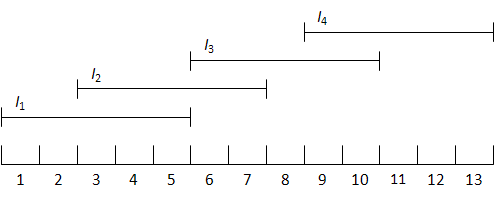For each interval i (i=1, . . . , I) there is a function gi representing the service level as a function of the number of employees working in that interval. Let yk be the number of employees of shift k for k = 1, . . . ,K. Then the number of employees hi(y) working at interval i is given by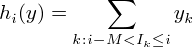The value of gi at this point is the attained service level in interval i. The overall service level is defined byNow consider the following problem, which maximizes the service level for a given number of employees: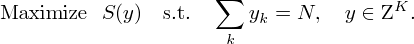We assume that call arrivals are Poisson. The service criterion is the fraction of customers that has to wait longer than c seconds, e.g., c=11 seconds, before getting an operator, which should be below 5% in general. (We assume that no customers leave the system before getting an operator.)

Assuming that a statistical equilibrium is attained in each time interval, we use the formula for the stationary waiting time in an M|M|n queue as the service level. Let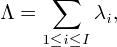then the expected service level of an arbitrary customer under schedule y is equal towhere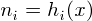and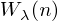are the waiting time in an M|M|n queue with arrival rate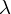and service rate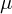. Thus we take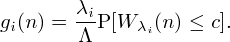This function is indeed monotone increasing and concave for each i. Therefore,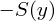is multimodular. We can minimizeby ODICON because multimodular functions and L-convex functions are equivalent objects that can be related through a simple coordinate transformation.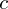: Service criterion (We measure the fraction of customers that has to wait longer thanseconds before getting an operator)= (seconds): Service rate per hour=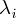: Arrival rates per hour, 0 <(= 1, 2, ... ,13)1 2 3 4 5 6 7 8 9 10 11 12 13y1 y2 y3 y4

You can input parametersin the ranges 0 < c < 20 and,in the ranges 0 <,< 100.

You can also input an initial solution y. The input should satisfy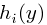>for all i. Then,

N = y1+y2+y3+y4 = .

(Note: "Reset" button resets your inputs to default values.)

This web application minimizes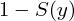using ODICON .

 G. Koole and E. van der Sluis (2003): Optimal shift scheduling with a global service level constraint, IIE Transactions, Vol. 35, pp. 1049-1055.